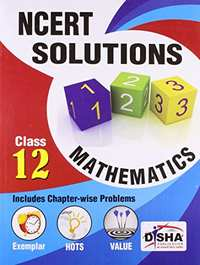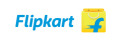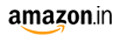Share

# NCERT Solutions with Exemplar/HOTS/Value based Questions: Class 12 - PCM (Set of 3 books) CBSE (Science) Class 12

SubjectNCERT Solutions with Exemplar/HOTS/Value based Questions: Class 12 - PCM (Set of 3 books)By Disha Experts (Author)
M.R.P 625/-335.00(46%)350.00(44%)
Product Description

The book NCERT Solutions Class 12 Physics, Chemistry and Mathematics (a set of 3 books) is exclusively written for CBSE students of class 12.

• The book provides Quick Revision of the concepts involved along with important formulas and definitions, in each chapter, which would act as a refresher.
• This is followed by the detailed solutions (Question-by-Question) of all the questions/exercises provided in the NCERT book for the current session.
• All the in-chapter questions of the NCERT books, wherever found, have been fully solved.
• The solutions have been designed in such a manner (Step-by-Step) that it would bring 100% Concept Clarity for the students.
• The solutions are Complete (each and every question is solved), Inflow (exactly on the flow of questions in the NCERT book) and Correct (Errorless).
• This set of books is a must for all class 12 appearing students.

Book 1 - Physics

• Electric Charges, Fields
• Electrostatic Potential and Capacitance
• Current Electricity
• Moving Charges and Magnetism
• Magnetism and Matter
• Electromagnetic Induction
• Alternating Current
• Electromagnetic Waves
• Light - Reflection and Refraction
• Wave Optics
• Dual Nature of Radiation and Matter
• Atoms
• Nuclei
• Semiconductor Electronics
• Communication Systems

Book 2 - Chemistry

• The Solid State
• Solutions
• Electrochemistry
• Chemical Kinetics
• Surface Chemistry
• General Principles and Processes of Isolation of Elements
• The p-block Elements (Group 15, 16, 17 and 18)
• The d- and f- block Elements
• Coordination Compounds
• Haloalkanes and Haloarenes
• Alcohols, Phenols and Ethers
• Aldehydes, Ketones and Carboxylic Acids
• Amines
• Biomolecules
• Polymers
• Chemistry in Everyday Life

Book 3 - Mathematics

• Relations and Functions
• Inverse Trigonometric Functions
• Matrices
• Determinants
• Continuity and Differentiability
• Application Of Derivatives
• Integrals
• Application Of Integrals
• Differential Equation
• Vector Algebra
• Three Dimensional Geometry
• Linear Programming
• Probability

S